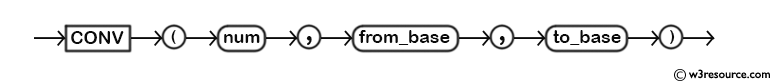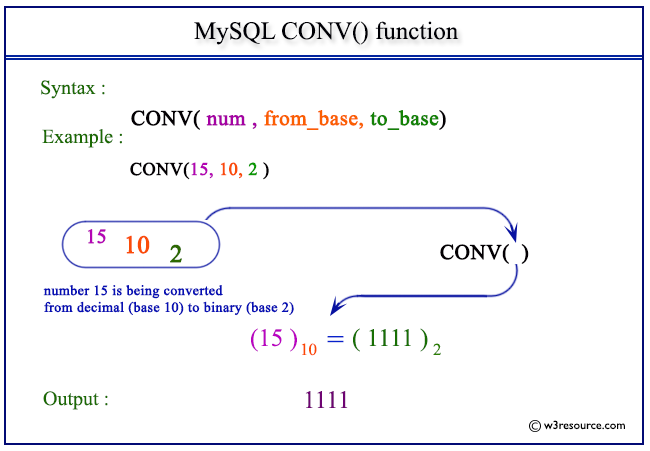# MySQL CONV() function

## CONV() function

MySQL CONV() converts a number from one numeric base number system to another numeric base number system. After the conversion, the function returns a string representation of the number.

When the argument defined is a NULL, the return value will be NULL.

The minimum base is 2 and the maximum base is 36. If the base to be converted to is a negative number, the number is regarded as a signed number. Otherwise, it is treated as unsigned.

Syntax:

```CONV(num , from_base , to_base );
```

Arguments

Name Description
num A number.
from_base Existing base of the number num.
to_base Base of the number num after conversion.

Syntax Diagram:MySQL Version: 5.6

Pictorial presentation of MySQL CONV() functionExample of MySQL CONV() function

Code:

``````SELECT CONV(15,10,2);
```
```

Explanation

The above MySQL statement will convert the numeric value of 15 from decimal number system to binary number system.

Sample Output:

```mysql> SELECT CONV(15,10,2);
+---------------+
| CONV(15,10,2) |
+---------------+
| 1111          |
+---------------+
1 row in set (0.02 sec)
```

Example : CONV() function using character value

Code:

``````SELECT CONV('b',16,10)'Hexadecimal to Decimal',
```
```

Explanation

The above MySQL statement will convert the hexadecimal ‘b’ in decimal number and binary number.

Sample Output:

```mysql> SELECT CONV('b',16,10)'Hexadecimal to Decimal',
->   CONV('b',16,2) AS 'Hexadecimal to Binary';
+------------------------+-----------------------+
+------------------------+-----------------------+
| 11                     | 1011                  |
+------------------------+-----------------------+
1 row in set (0.02 sec)
```

Example : CONV() function using negative base

Code:

``````SELECT CONV(19,10,-16);
```
```

Explanation

The above MySQL statement will convert a decimal value 19 to a hexadecimal number. Here the base to be converted is -16, so it is treated as an unsigned number.

Sample Output:

```mysql> SELECT CONV(19,10,-16);
+-----------------+
| CONV(19,10,-16) |
+-----------------+
| 13              |
+-----------------+
1 row in set (0.00 sec)
```

All Mathematical FunctionsPrevious: CEILING()
Next: COS()

﻿